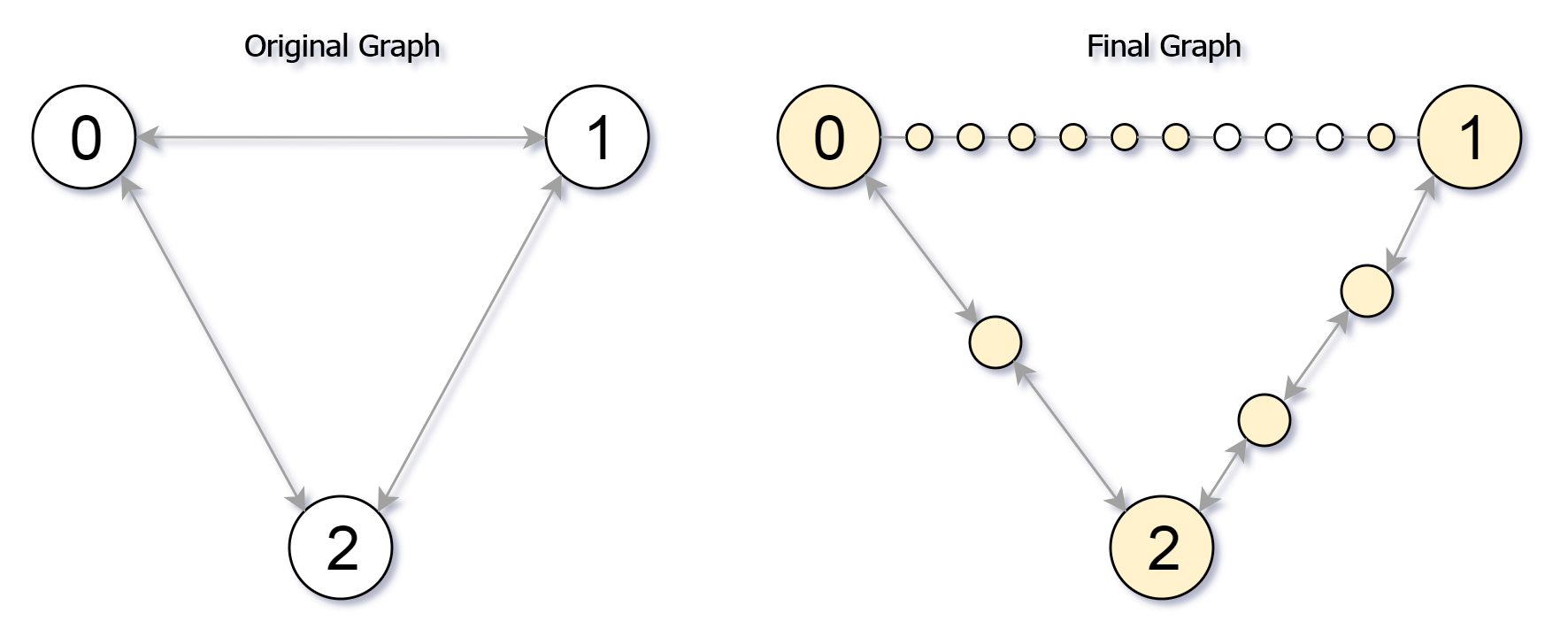Formatted question description: https://leetcode.ca/all/882.html

# 882. Reachable Nodes In Subdivided Graph (Hard)

Starting with an undirected graph (the "original graph") with nodes from 0 to N-1, subdivisions are made to some of the edges.

The graph is given as follows: edges[k] is a list of integer pairs (i, j, n) such that (i, j) is an edge of the original graph,

and n is the total number of new nodes on that edge.

Then, the edge (i, j) is deleted from the original graph, n new nodes (x_1, x_2, ..., x_n) are added to the original graph,

and n+1 new edges (i, x_1), (x_1, x_2), (x_2, x_3), ..., (x_{n-1}, x_n), (x_n, j) are added to the original graph.

Now, you start at node 0 from the original graph, and in each move, you travel along one edge.

Return how many nodes you can reach in at most M moves.

Example 1:

Input: edges = [[0,1,10],[0,2,1],[1,2,2]], M = 6, N = 3
Output: 13
Explanation:
The nodes that are reachable in the final graph after M = 6 moves are indicated below.Example 2:

Input: edges = [[0,1,4],[1,2,6],[0,2,8],[1,3,1]], M = 10, N = 4
Output: 23

Note:

1. 0 <= edges.length <= 10000
2. 0 <= edges[i] < edges[i] < N
3. There does not exist any i != j for which edges[i] == edges[j] and edges[i] == edges[j].
4. The original graph has no parallel edges.
5. 0 <= edges[i] <= 10000
6. 0 <= M <= 10^9
7. 1 <= N <= 3000
8. A reachable node is a node that can be travelled to using at most M moves starting from node 0.

Related Topics:
Heap

## Solution 1. Dijkstra

// OJ: https://leetcode.com/problems/reachable-nodes-in-subdivided-graph/
// Time: O(ElogN)
// Space: O(N)
// Ref: https://leetcode.com/problems/reachable-nodes-in-subdivided-graph/solution/
class Solution {
typedef pair<int, int> pii;
public:
int reachableNodes(vector<vector<int>>& edges, int M, int N) {
vector<vector<pii>> G(N);
for (auto &e : edges) {
int u = e, v = e, w = e;
G[u].emplace_back(v, w);
G[v].emplace_back(u, w);
}
map<int, int> dist;
dist = 0;
for (int i = 1; i < N; ++i) dist[i] = M + 1; // M is the maximum steps we can take, so M + 1 is the smallest invalid value.
map<pii, int> used;
int ans = 0;
priority_queue<pii, vector<pii>, greater<pii>> q; // weight, node
q.emplace(0, 0);
while (q.size()) {
auto top = q.top();
q.pop();
int d = top.first, u = top.second;
if (d > dist[u]) continue;
++ans;
for (auto [v, w] : G[u]) {
used[{ u, v }] = min(w, M - d); // M - d is how much further we can walk from this u node
int d2 = d + w + 1; // d2 is the minimal distance from source node to this v node.
if (d2 < min(dist[v], M + 1)) {
q.emplace(d2, v);
dist[v] = d2;
}
}
}
for (auto &e : edges) {
int u = e, v = e, w = e;
ans += min(w, used[{ u, v }] + used[{ v, u }]);
}
return ans;
}
};


Java

• class Solution {
public int reachableNodes(int[][] edges, int M, int N) {
Map<Integer, Map<Integer, Integer>> graphMap = new HashMap<Integer, Map<Integer, Integer>>();
for (int[] edge : edges) {
int node0 = edge, node1 = edge, insertions = edge;
Map<Integer, Integer> map0 = graphMap.getOrDefault(node0, new HashMap<Integer, Integer>());
Map<Integer, Integer> map1 = graphMap.getOrDefault(node1, new HashMap<Integer, Integer>());
map0.put(node1, insertions);
map1.put(node0, insertions);
graphMap.put(node0, map0);
graphMap.put(node1, map1);
}
int[] distances = new int[N];
Arrays.fill(distances, M + 1);
distances = 0;
int[][] used = new int[N][N];
int reachable = 0;
PriorityQueue<int[]> priorityQueue = new PriorityQueue<int[]>(new Comparator<int[]>() {
public int compare(int[] nodeDistance1, int[] nodeDistance2) {
return nodeDistance1 - nodeDistance2;
}
});
priorityQueue.offer(new int[]{0, 0});
while (!priorityQueue.isEmpty()) {
int[] nodeDistance = priorityQueue.poll();
int node = nodeDistance, distance = nodeDistance;
if (distance > distances[node])
continue;
reachable++;
if (graphMap.containsKey(node)) {
Map<Integer, Integer> edgesMap = graphMap.get(node);
Set<Integer> keySet = edgesMap.keySet();
for (int adjacent : keySet) {
int currReachable = Math.min(insertions, M - distance);
int newDistance = distance + insertions + 1;
}
}
}
}
for (int[] edge : edges) {
int node0 = edge, node1 = edge, insertions = edge;
reachable += Math.min(insertions, used[node0][node1] + used[node1][node0]);
}
return reachable;
}
}

• // OJ: https://leetcode.com/problems/reachable-nodes-in-subdivided-graph/
// Time: O(ElogE)
// Space: O(E)
// Ref: https://leetcode.com/problems/reachable-nodes-in-subdivided-graph/solution/
class Solution {
public:
int reachableNodes(vector<vector<int>>& E, int maxMoves, int n) {
vector<vector<pair<int, int>>> G(n);
for (auto &e : E) {
int u = e, v = e, w = e;
G[u].emplace_back(v, w);
G[v].emplace_back(u, w);
}
vector<int> step(n, -1);
step = maxMoves;
priority_queue<pair<int, int>> pq; // Max heap. Each entry is (stepsLeft, nodeIndex)
pq.emplace(maxMoves, 0);
int ans = 0;
while (pq.size()) {
auto [stepsLeft, u] = pq.top();
pq.pop();
if (stepsLeft < step[u]) continue;
++ans;
if (stepsLeft == 0) continue;
for (auto &[v, w] : G[u]) {
if (step[v] < stepsLeft - w - 1) {
step[v] = stepsLeft - w - 1;
pq.emplace(step[v], v);
}
}
}
for (auto &e : E) ans += min(e, max(0, step[e]) + max(0, step[e]));
return ans;
}
};

• print("Todo!")